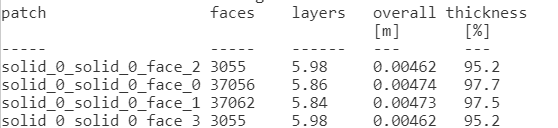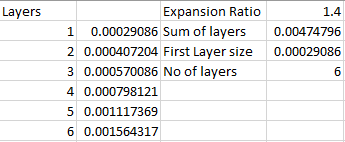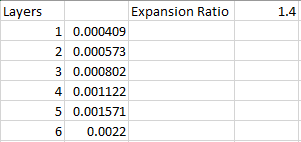# YPlus Wall Spacing Airfoil

Great post here @jousefm!

I made a simple simulation of an airfoil just to investigate if I could get a good Y-plus value after reading this post.

Mesh

• Airfoil with 1 meter chord length
• Background mesh box = 7x3x3 m

Simulation settings

• Turbulence model = k-omega SST
• Free stream velocity =1 m/s

Calculation of first layer thickness
I used this site: http://www.pointwise.com/yplus/, to calculate the wall spacing.
Here is what I put in to the calculator:

So the first layer should be 0.00029 m.

If I have 6 inflate boundary layers and an expansion ratio of 1.4, this gives me the following final layer thickness:

0.00029*1.4^(6-1)=0.0016 m

and the total thickness of the inflate boundary layers would be 0.00029*1.4^0+0.00029*1.4^1+0.00029*1.4^2+0.00029*1.4^3+
0.00029*1.4^4+0.00029*1.4^5=0.0047 m

The overall thickness from the meslog seems th correspond well with the calculated value:So after I made the simulation I imported the result into paraview. This is how it looks:

As you can see, the Y-plus seems to be below 0.05 for most of the wings surface. Is it normal to have so big difference between the estimated value and the actual value?

Hi @Filiptheking and sorry for the delayed feedback.

Will have a look at this and either @Get_Barried or me will get back to you!

Cheers!

Jousef

1 Like

The layers look good so far, but you will see some interesting problems as I describe below. Namely how ParaView shows y+ is less than 1 but it actually isn’t.

The differences should be minute due to possible rounding errors as you would expect. However, I’ve recalculated the layer sizes from the data you provided and got this.You have set your final layer size to 0.0022 which is slightly bigger than what would be your upper limit of the layer size.

From your input value of 0.0022, I’ve re-calculated backwards the size of the first layer and you can see below that the first cell size does correspond with the actual layer size that I’ve measured in ParaView.I suggest trying with the properly calculated set of values shown earlier and see if ParaView is able to show a y+ that is closer to expected margin.

I would also suggest performing a backwards calculation to ensure y+ is met. I have not been able to find documentation on how exactly does ParaView or the result control obtain its y+ value to be shown and it is true that there seems to be a large discrepancy in the displayed y+ value.

With that being said, for practical purposes all you have to do is to ensure the first layer cell height matches the calculated one and that the y+ is within your specified margins. In this case, ParaView shows that it is within margin but yet, it actually is not as the calculated first layer height is smaller than the current layer height (which can be fixed by following the first table of calculations).

Cheers.

Regards,
Barry

3 Likes

Yes, I can see that I made some errors.
However, in the mesh that is named “Y+ Experiment 2”, I did put in the correct final wall thickness of 0.0016.
I should have removed the first mesh, but I forgot it, sorry for that. Also, I used the first mesh with the final layer height of 0.0022 in both simulations, which was a mistake (I have fixed that now)

When I measure in the mesh the first layer is approximately 0.0016, which corresponds well with the calculated value. I’ve also done a backwards calculation with a first wall thickness of 0.00029, it gives me a Y+ of approximately 1.

However, the main problem is that the Y+ obtained from the result control item is so far from the expected value of 1.

This would be interesting to know!

Sincerely
Filip

1 Like